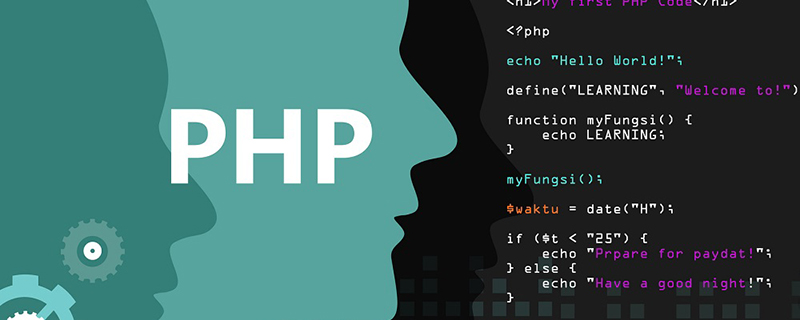# 如何解决php ajax乱码问题

php ajax乱码的解决办法：1、将所有数据统一使用utf-8编码；2、使用“JSON”和“arrayRecursive”两个函数，对每个字符分别编码。php使用ajax中文乱码问题

php数组：

```\$gg=array('a'=>'中国','b'=>'日本','c'=>'美国','d'=>'加拿大','e'=>'法国');
\$yy=array('f'=>'6','g'=>'7','h'>='8','i'=>'tom','j'=>'kevin');```

`echo \$json=json_encode(\$gg);`

`{"a":"\u4e2d\u56fd","b":"\u65e5\u672c","c":"\u7f8e\u56fd","d":"\u52a0\u62ff\u5927","e":"\u6cd5\u56fd"}`

`echo \$json=json_encode(array(\$gg,\$yy));`

`[{"a":"\u4e2d\u56fd","b":"\u65e5\u672c","c":"\u7f8e\u56fd","d":"\u52a0\u62ff\u5927","e":"\u6cd5\u56fd"},{"f":"6","g":"7","0":true,"i":"tom","j":"kevin"}]`

```function JSON(\$array) {
arrayRecursive(\$array, 'urlencode', true);
\$json = json_encode(\$array);
return urldecode(\$json);
}
function arrayRecursive(&\$array, \$function, \$apply_to_keys_also = false){
static \$recursive_counter = 0;
if (++\$recursive_counter > 1000) {
die('possible deep recursion attack');
}
foreach (\$array as \$key => \$value) {
if (is_array(\$value)) {
arrayRecursive(\$array[\$key], \$function, \$apply_to_keys_also);
} else {
\$array[\$key] = \$function(\$value);
}
if (\$apply_to_keys_also && is_string(\$key)) {
\$new_key = \$function(\$key);
if (\$new_key != \$key) {
\$array[\$new_key] = \$array[\$key];
unset(\$array[\$key]);
}
}
}
\$recursive_counter--;
}```

`[{"a":"中国","b":"日本","c":"美国","d":"加拿大","e":"法国"},{"f":"6","g":"7","0":"1","i":"tom","j":"kevin"}]`#### 相关文章推荐

• javascript如何实现ajax• php ajax提交中文乱码怎么办• ajax请求报错，php如何开启跨域请求• 继续学习——AJAX PHP（附源码）

### 全部评论我要评论

• 取消发布评论发送
• 1/1